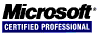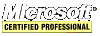# .NET C# Guide

## Classes

A class is a template that defines the form of an object. It specifies the code (methods and properties) that will operate on its data. Objects are instances of a class.

### Defining a Class

To allow outside code to access the class data, use the `public` access modifier. This is a very simple class definition and is in no way complete:

``````class Rectangle
{
public int width, height;
public string name;
}
``````

#### Creating Objects

Objects are created by using the name of the non-static class as the type and the `new` operator. It's as easy as one simple statement:

``Rectangle rect = new Rectangle();``

#### Static Classes

Static classes can not be instantiated.

``````static class Rectangle
{
public static int width, height;
public static string name;
}
``````

### Methods/Functions

Methods are subroutines that may manipulate the data and/or perform calculations on it. A method can return data or nothing in which case it should have a return type of void. An example of defining a method (`Area()`) is here:

``````class Rectangle
{
public int width, height;
public string name;

public int Area() // return type is int
{
// return the area of the rectangle
return width * height;
}
}
``````

#### Using Parameters

The value passed to a method is called an argument. From the code inside the method, the variable that recieved the argument is known as a parameter. The `SetWidthHeight()` method here uses parameters:

``````class Rectangle
{
public int width, height;
public string name;

public int Area()
{
// return the area
return width * height;
}

// void means it does not return a value
public void SetWidthHeight(int x, int y)
{
width = x;
height = y;
}
}
``````

#### ref and out parameters

Use `ref` and `out` to pass any of the normal types (int, string, double, etc.) by reference. The difference is that when using `ref` the parameter must be initialized with a value because it is for both input and output. `out` does not require the variable be initialized. It's for output only.

``````class Rectangle
{
public int width, height;
public string name;

public int Area()
{
return width * height;
}

public void GetWidthHeight(out int x, out int y)
{
x = width;
y = height;
}

public void SetWidthHeight(int x, int y)
{
width = x;
height = y;
}
}
``````

Arrays are technically passed by reference but if needing to assign a new array to the identifier then it will have to be passed by reference.

``````class ArrayByReferenceOrValue
{
public void PassByValue(char[] chars)
{
chars = 'a'; // this will change the array for the caller
chars = new char; // this will do nothing
}
public void PassByReference(ref char[] chars)
{
chars = new char; // this will change the array for the caller
}
}
``````

#### Variable Number of Arguments

It is possible for a method to have an arbitrary number of arguments using the `params` modifier and specifying an array for the parameter.

Overloading is when two or more methods in the class share the same name but have different parameters. They can not be defined exactly the same.

``````class Rectangle
{
public int width, height;
public string name;

public int Area()
{
return width * height;
}

public void SetWidthHeight(int x, int y)
{
width = x;
height = y;
}

public void SetWidthHeight(int x)
{
width = height = x;
}
}
``````

#### static Methods

When a member is declared static, it can be accessed before any objects of the class are instantiated and without reference to any object.

``````class Rectangle
{
public int width, height;
public string name;

public int Area()
{
return width * height;
}

// can be used as Rectangle.Area(w, h)
public static int Area(int w, int h)
{
// this method can not access width, height, or
// name because it is not accessed through an object
// but it can access static data members
return w * h;
}

public void SetWidthHeight(int x, int y)
{
width = x;
height = y;
}
}
``````

#### Lambda Expressions

``````static void Main()
{
// here a lambda returns a string value
Func<string> getAString = () =>
{
return "some string";
};
Console.WriteLine(getAString());

// here a lambda returns a double value and accepts two double parameters
Func<double, double, double> addTwoNumbers = (num1, num2) =>
{
return num1 + num2;
};
}
``````

### Constructors

The constructor initializes an object upon creation. It must have the same name as the class and not define a return type (not even `void`) and it should use the `public` access modifier. The `private` access modifier is introduced here and when a member is specified as `private` then only other members of the class have access to it.

``````class Rectangle
{
// notice that these data members are not public
private int width, height;
private string name;

// constructor
public Rectangle()
{
// initialize to one cubic unit of measure
width = height = 1;
}

public int Area()
{
// return the area
return width * height;
}

public void SetWidthHeight(int x, int y)
{
width = x;
height = y;
}
}
``````

#### Parameterized Constructors

With a parameterized constructor, data member variables are set to the values of parameters.

``````class Rectangle
{
private int width, height;
private string name;

public Rectangle()
{
width = height = 1;
}

// parameterized constructor
public Rectangle(int x, int y)
{
width = x;
height = y;
}

public int Area()
{
// return the area
return width * height;
}

public void SetWidthHeight(int x, int y)
{
width = x;
height = y;
}
}
``````

### Destructors

Generally, destructors are not needed because C# automatically deallocates any memory used by the object, but should there be a reason (perhaps to close connections or files) then a destructor can be defined.

``````class Rectangle
{
private int width, height;
private string name;

public Rectangle()
{
width = height = 1;
}

public Rectangle(int x, int y)
{
width = x;
height = y;
}

// destructor
~Rectangle()
{
// do something
}

public int Area()
{
// return the area
return width * height;
}

public void SetWidthHeight(int x, int y)
{
width = x;
height = y;
}
}
``````

### this

The `this` keyword refers to the object itself from within its own code.

``````class Rectangle
{
private int width, height;
private string name;

public Rectangle()
{
this.width = this.height = 1;
}

public Rectangle(int x, int y)
{
this.width = x;
this.height = y;
}

// destructor
~Rectangle()
{
// do something
}

public int Area()
{
// return the area
return this.width * this.height;
}

public void SetWidthHeight(int x, int y)
{
this.width = x;
this.height = y;
}
}
``````

### Properties

The property is another type of class member. It combines a data member with the methods that access it. It uses the `get` and `set` accessors. The `set` accessor receives a parameter named `value`.

``````class Rectangle
{
private int width, height;
private string name;

public Rectangle()
{
Width = Height = 1;
}

public Rectangle(int x, int y)
{
Width = x;
Height = y;
}

~Rectangle()
{
// do something
}

public int Area()
{
return Width * Height;
}

// Width property
public int Width
{
get
{
return width;
}
set
{
if(value > 0)
width = value;
}
}

// Height property
public int Height
{
get
{
return height;
}
set
{
if(value > 0)
height = value;
}
}
}
``````
<< < [Page 5 of 7] > >>